# Bubble Sort in Python Programming

###### Sorting in Python

In this lesson, we will understand what is Bubble Sort in Python Programming along with some examples.

## What is Bubble Sort in Python?

A Bubble Sort is a sorting technique used in python to sort elements of sequences like arrays and lists in ascending or descending order.

In this sorting technique, elements are sorted in ascending order by repeatedly swapping the larger element with the smaller element. While in descending order, sorting is performed by repeatedly swapping the smaller element with the larger element.

To clearly understand the bubble sorting technique, let's go through its algorithm step by step with an example.## Bubble Sort Visualization

Suppose we have seven numbers stored in a list as shown below.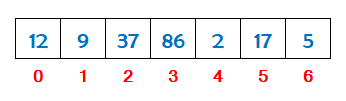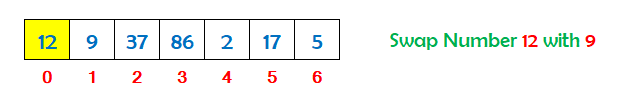Take the 1st number 12 and compare it with the 2nd number 9. 12 is greater than 9 so swap both the numbers.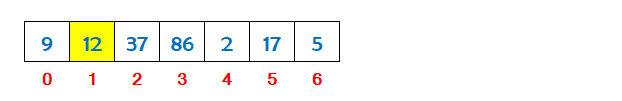Now take the 2nd number 12 and compare it with the 3rd number 37. 12 is not greater than 37 so leave it.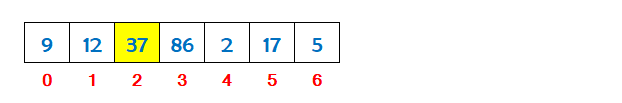Now take the 3rd number 37 and compare it with the 4th number 86. 37 is not greater than 86 so leave it.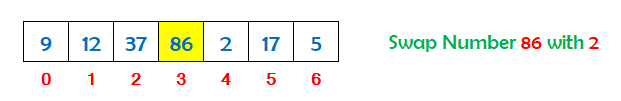Now take the 4th number 86 and compare it with the 5th number 2. 86 is greater than 2 so swap both the numbers.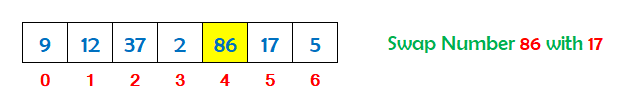Now take the 5th number 86 and compare it with the 6th number 17. 86 is greater than 17 so swap both the numbers.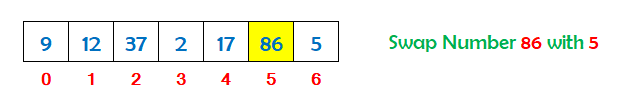Now take the 6th number 86 and compare it with the 7th number 5. 86 is greater than 5 so swap both the numbers.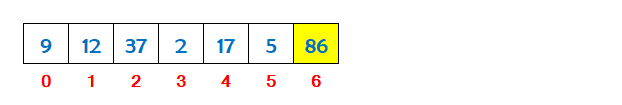So we can see that the largest number has shifted towards the end of the list. To arrange the other numbers also, we have to repeat the above process five times more from index 0 to index 4.

If there are ten number in a list, we have to repeat the above process nine times to sort the entire list.

## Algorithm for Bubble Sort

An algorithm is the steps used to implement the bubble sort in a python program.

Assume we have N numbers stored in a list. To implement the bubble sort on N numbers, the steps are as follows.

• Define a list to store N numbers for bubble sort. Suppose we have defined a list with the name num.
• Run an outer loop i from 0 to N-1 to repeat the process of bubble sort.
• Run an inner loop j inside the body of the outer loop i for bubble sort from 0 to N-1-i.
• Inside the body of the inner loop j, check if the value at num[j] is greater than the value at num[j+1].
• If the value at num[j] is greater than value at num[j+1] then swap the numbers.
• Now print the sorted list on the screen outside the body of the outer loop i.

## Python Program for Bubble Sort

A Python program to sort a list of numbers in ascending order using the bubble sort technique.

#### Bubble Sort (List of Numbers in Ascending Order)

``````# define a list
num=[12,9,37,86,2,17,5]

print('List before Bubble Sort')
for n in num:
print(n,end=' ')

# run an outer loop i from 0 to len(num)-1 to repeat the process of bubble sort
for i in range(0,len(num)-1):

# run an inner loop j for bubble sort from 0 to len(num)-1-i
for j in range(0,len(num)-1-i):

# now check if the value at num[j] is greater than value at num[j+1]
if num[j]>num[j+1]:
# if the value is greater then swap the numbers
t=num[j]
num[j]=num[j+1]
num[j+1]=t

# print the sorted list
print('\n\nList after Bubble Sort')
for n in num:
print(n,end=' ')``````

#### Output

```List before Bubble Sort
12 9 37 86 2 17 5

List after Bubble Sort
2 5 9 12 17 37 86```

A Python program to sort a list of numbers in descending order using the bubble sort technique.

#### Bubble Sort (List of Numbers in Descending Order)

``````# define a list
num=[12,9,37,86,2,17,5]

print('List before Bubble Sort')
for n in num:
print(n,end=' ')

# run an outer loop i from 0 to len(num)-1 to repeat the process of bubble sort
for i in range(0,len(num)-1):

# run an inner loop j for bubble sort from 0 to len(num)-1-i
for j in range(0,len(num)-1-i):

# now check if the value at num[j] is smaller than value at num[j+1]
if num[j]<num[j+1]:
# if the value is smaller then swap the numbers
t=num[j]
num[j]=num[j+1]
num[j+1]=t

# print the sorted list
print('\n\nList after Bubble Sort')
for n in num:
print(n,end=' ')``````

#### Output

```List before Bubble Sort
12 9 37 86 2 17 5

List after Bubble Sort
86 37 17 12 9 5 2```

A Python program to sort a list of strings in ascending order using the bubble sort technique.

#### Bubble Sort (List of Strings in Ascending Order)

``````# define a list
num=['Squirrel','Dog','Panda','Lion','Bear','Tiger','Rabbit']

print('List before Bubble Sort')
for n in num:
print(n,end='  ')

# run an outer loop i from 0 to len(num)-1 to repeat the process of bubble sort
for i in range(0,len(num)-1):

# run an inner loop j for bubble sort from 0 to len(num)-1-i
for j in range(0,len(num)-1-i):

# now check if the value at num[j] is greater than value at num[j+1]
if num[j]>num[j+1]:
# if the value is greater then swap the strings
t=num[j]
num[j]=num[j+1]
num[j+1]=t

# print the sorted list
print('\n\nList after Bubble Sort')
for n in num:
print(n,end='  ')``````

#### Output

```List before Bubble Sort
Squirrel  Dog  Panda  Lion  Bear  Tiger  Rabbit

List after Bubble Sort
Bear  Dog  Lion  Panda  Rabbit  Squirrel  Tiger```

## Bubble Sort Program Explanation

The explanation of the above program (List of Numbers in Ascending Order) is given below in details.

#### Explanation

```List before Bubble Sort
12 9 37 86 2 17 5

The outer loop i starts running from 0 to 5

When i = 0
The inner loop j starts running from 0 to 5

When j = 0
List is : 12 9 37 86 2 17 5    Check if 12>9   Yes 12>9 swap the numbers
List is : 9 12 37 86 2 17 5

When j = 1
List is : 9 12 37 86 2 17 5    Check if 12>37   No

When j = 2
List is : 9 12 37 86 2 17 5    Check if 37>86   No

When j = 3
List is : 9 12 37 86 2 17 5    Check if 86>2   Yes 86>2 swap the numbers
List is : 9 12 37 2 86 17 5

When j = 4
List is : 9 12 37 2 86 17 5    Check if 86>17   Yes 86>17 swap the numbers
List is : 9 12 37 2 17 86 5

When j = 5
List is : 9 12 37 2 17 86 5    Check if 86>5   Yes 86>5 swap the numbers
List is : 9 12 37 2 17 5 86

When i = 1
The inner loop j starts running from 0 to 4

When j = 0
List is : 9 12 37 2 17 5 86    Check if 9>12   No

When j = 1
List is : 9 12 37 2 17 5 86    Check if 12>37   No

When j = 2
List is : 9 12 37 2 17 5 86    Check if 37>2   Yes 37>2 swap the numbers
List is : 9 12 2 37 17 5 86

When j = 3
List is : 9 12 2 37 17 5 86    Check if 37>17   Yes 37>17 swap the numbers
List is : 9 12 2 17 37 5 86

When j = 4
List is : 9 12 2 17 37 5 86    Check if 37>5   Yes 37>5 swap the numbers
List is : 9 12 2 17 5 37 86

When i = 2
The inner loop j starts running from 0 to 3

When j = 0
List is : 9 12 2 17 5 37 86    Check if 9>12   No

When j = 1
List is : 9 12 2 17 5 37 86    Check if 12>2   Yes 12>2 swap the numbers
List is : 9 2 12 17 5 37 86

When j = 2
List is : 9 2 12 17 5 37 86    Check if 12>17   No

When j = 3
List is : 9 2 12 17 5 37 86    Check if 17>5   Yes 17>5 swap the numbers
List is : 9 2 12 5 17 37 86

When i = 3
The inner loop j starts running from 0 to 2

When j = 0
List is : 9 2 12 5 17 37 86    Check if 9>2   Yes 9>2 swap the numbers
List is : 2 9 12 5 17 37 86

When j = 1
List is : 2 9 12 5 17 37 86    Check if 9>12   No

When j = 2
List is : 2 9 12 5 17 37 86    Check if 12>5   Yes 12>5 swap the numbers
List is : 2 9 5 12 17 37 86

When i = 4
The inner loop j starts running from 0 to 1

When j = 0
List is : 2 9 5 12 17 37 86    Check if 2>9   No

When j = 1
List is : 2 9 5 12 17 37 86    Check if 9>5   Yes 9>5 swap the numbers
List is : 2 5 9 12 17 37 86

When i = 5
The inner loop j starts running from 0 to 0

When j = 0
List is : 2 5 9 12 17 37 86    Check if 2>5   No

The outer loop ends

List after Bubble Sort
2 5 9 12 17 37 86```